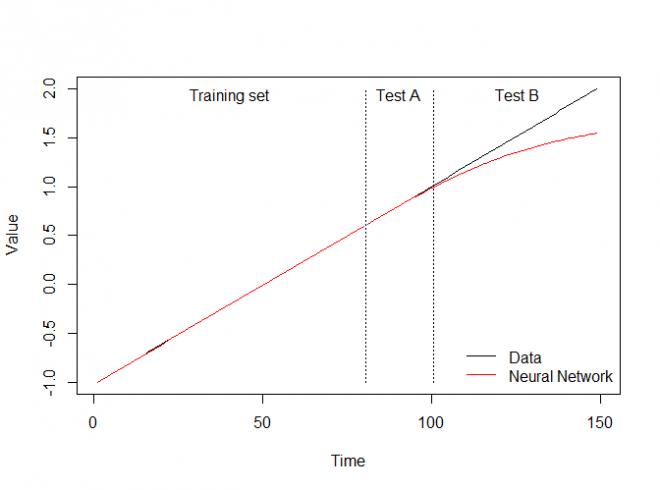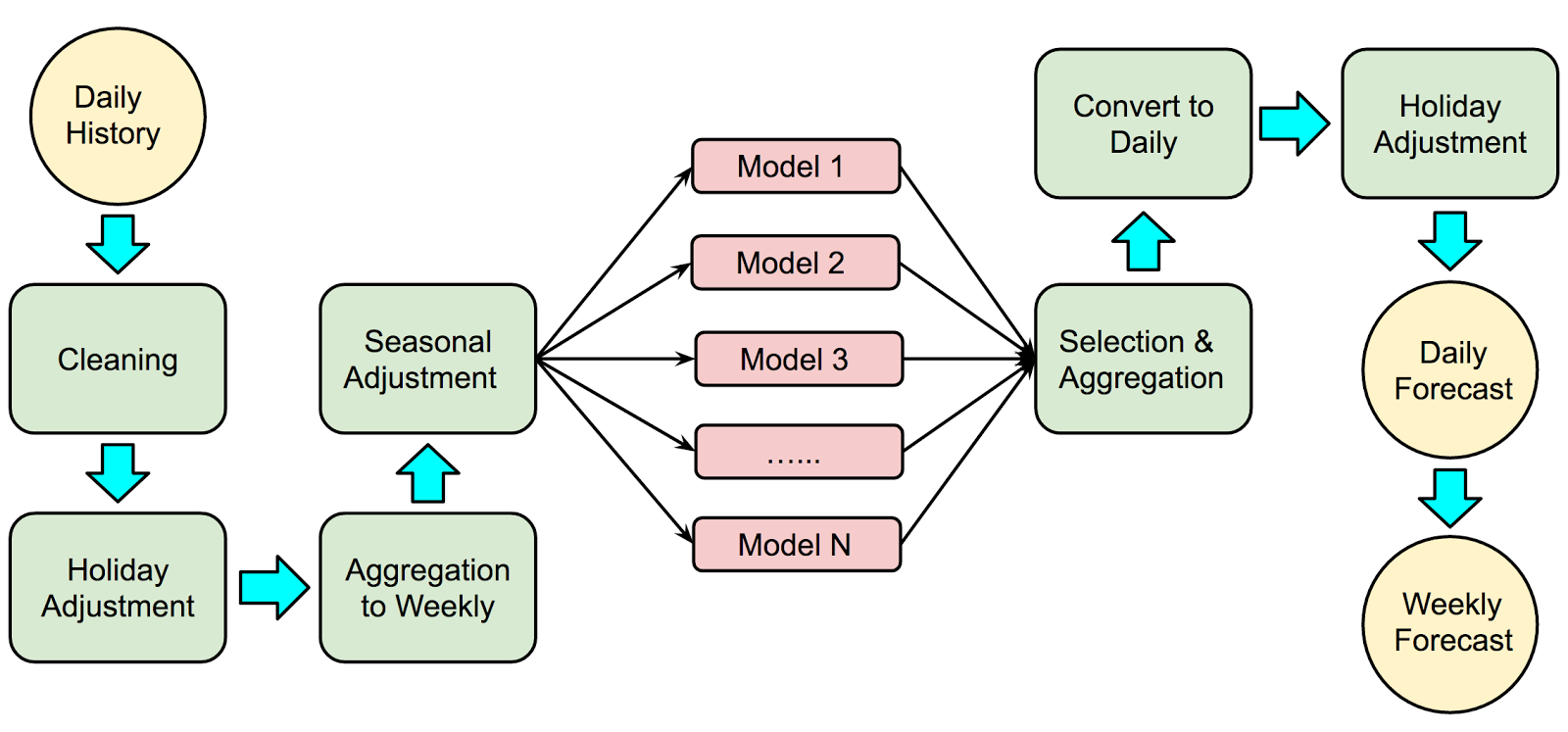# ARIMA vs. long term forecasts

## Anton Lebedevich:

• data science engineer
• solves distributed performance issues
• loves time series and anomalies
• blogs at mabrek.github.io
A time series is a collection of observations made sequentially in time.## Forecasting Challenges

• many seasons (year, month?, week, day)
• external predictors (weather, promo)
• data gaps
• outliers, changepoints
• holidays (zero values)
• irregular (sometimes)
• short train

## SARIMA

$$\phi_p (L) \tilde \phi_P (L^s) \Delta^d \Delta_s^D y_t = A(t) + \theta_q (L) \tilde \theta_Q (L^s) \zeta_t$$

## SARIMA

$$\phi_p (L) \tilde \phi_P (L^s) \Delta^d \Delta_s^D y_t = A(t) + \theta_q (L) \tilde \theta_Q (L^s) \zeta_t$$## ARIMA (simple form)

$$y'_{t} = c + \phi_{1}y'_{t-1} + \cdots + \phi_{p}y'_{t-p} \\ + \theta_{1}e_{t-1} + \cdots + \theta_{q}e_{t-q} + e_{t}$$## Dirac Delta Function

used to measure impulse response## AR(1) Impulse Response

$$y_{t} = 0.9y_{t-1} + e_{t}$$## AR(2) Impulse Response

$$y_{t} = 0.3y_{t-1} + 0.3y_{t-2} + e_{t}$$## AR(2) Impulse Response

$$y_{t} = 0.9y_{t-1} - 0.85y_{t-2} + e_{t}$$## AR(2) Impulse Response

$$y_{t} = 0.08y_{t-1} + 0.9y_{t-2} + e_{t}$$## I(0) Process (White Noise)

$$y_{t} \sim \mathcal{N}(0,1)$$## I(1) Process (Random Walk)

$$y_{t} = y_{t-1} + e_{t}$$## I(2) Process## Weekly Pattern + Noise## auto.arima## ARIMA(7, 0, 0)## SARIMAX Challenges

• - many seasons (year, month, week, day)
• + external predictors (weather, promo)
• - data gaps
• - outliers, changepoints
• - holidays (zero values)
• - irregular (sometimes)
• ÷ short train

• linear
• trees (XGBoost, etc..)
• combine models
• neural networks
• bayesian
• multiple output

## Naïve Methods

• just keep last value
• mean or median
• seasonal

## Linear Models

• lags
• categorical seasons
• Fourier seasons
• window aggregates
• holiday shoulders
• promo actionssimple average

## Neural Networks

• entity embeddings
• LSTM
• more layers## Bayesian

• BSTS
• PyDLM, PyFlux, PyMC3, PyStan

• Direct
• DirRec
• MIMO
• DIRMO

## Out-of-sample Extrapolationellisp.github.io/blog/2016/12/10/extrapolation

## NN doesn't Extrapolate Toohttp://kourentzes.com/forecasting/2016/12/28/can-neural-networks-predict-trended-time-series/

## Example Workflow## Competitions@widdoc